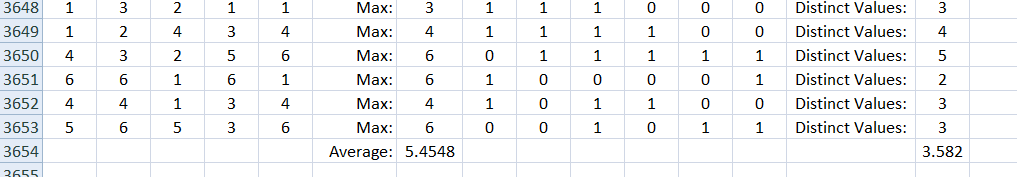# James Tanton is Still Rolling

And now he asks:

“If I roll a die five times, how many distinct values should I expect to see?”

I was feeling pretty good about myself having solved the first one. (Here) And my Excel simulation told me I was at least close.  But when I attacked this new one, I hit some trouble.  I know I need the expected values, but to get them, I need the probabilities.  And I was bogging down in the calculations.  I could find the probability of getting one distinct value, but two was harder, three harder still…

Simulate first and calculate later

I already had the spreadsheet with the random integers.  But Excel does not have a “number of discrete values on the list” function.  So even there, I was stuck.

[Side note: back in the day when I did know how to code, I briefly knew an obscure programming language called APL.  I believe that in APL, a problem like this can be solved in a single line of code, dense with obscure symbols.  Document your code, campers, or you will never remember what you did!]

But since JT only rolled the dice 5 times, I did eventually come up with a way to have Excel do this for me. Let’s call it the “Go Fish” procedure, naming it after the simple card game.  It’s not very elegant but it works and, as you will see, it pays extra dividends.

For each possible dice value, 1 through 6, I made a column that answered the question: did this value appear on the list. For example, the formula in my first column answers the Go Fish question: got any ones?

=1×or((a2=1),(b2=1),(c2=1),(d2=1),(e2=1))

This generates a value of 1 if any of the dice came up as a 1 and zero if none of them did.

I made a total of 6 columns like this. Then, the sum of those columns tells me how many distinct values appeared in my original 5 rolls of the dice.

From there, it was just a matter of doing this in every row and taking the average. Again I did 10 years worth of rows.You can see in the top row that the dice came up: 1, 3, 2, 1, 1

So the answer was yes if the question was got any 1’s, 2’s or 3’s and no for any 4’s, 5’s or 6’s.  That gave a total of 3 distinct values this time.

OK, so now I have a rough idea of the answer. But aren’t I just stalling?  I should get back to work calculating the probabilities and expected values.

[Really, I am stalling.  I should be grading lab reports.]

Then I realized that the procedure I used to generate the answer in the simulation can be used to calculate the answer directly:

Say you want to know the probability that your list contains a 1. That is more easily calculated as 1 minus the probability that it contains no 1’s.

P(got any ones) = 1 – (5/6)5

But that is also the probability for any of the single Go Fish questions:

P(got any ones) = P(got any twos) = P(got any threes)…and so on.

So the expected value of the total of the “Go Fish” questions = 6 times the value for any one of them!

That means we expect 6×(1 – (5/6)5) = 3.589 different results every time.

That’s not far from what the simulation told me to expect. I ran it 5 more times and got:

3.584, 3.591, 3.586, 3.587, 3.595

So I am feeling like this is a promising answer. I would still like to go back and finish calculating the probabilities the hard way.  But the point to notice here is that taking the time to simulate the problem also provided the key to a solution path.